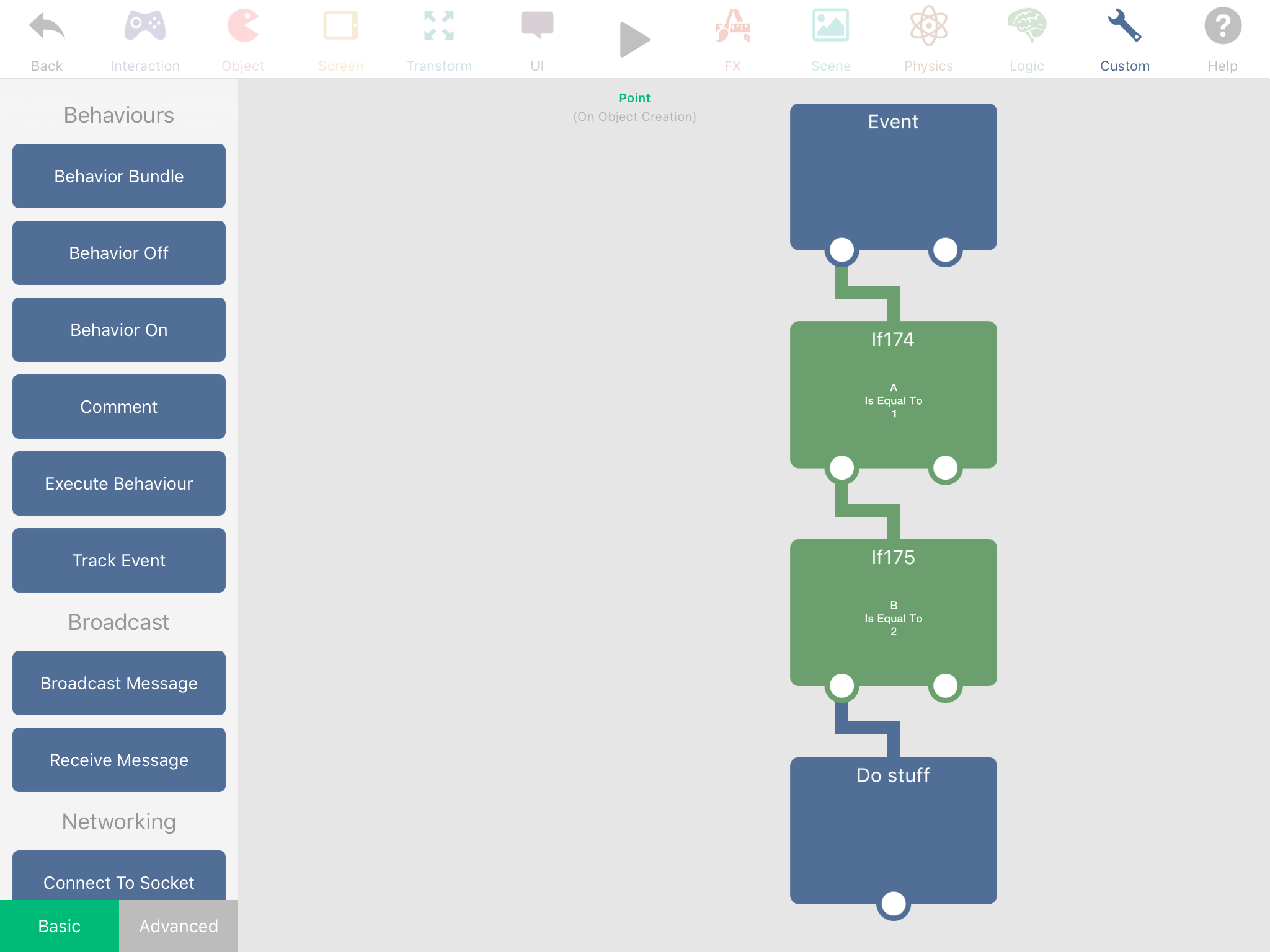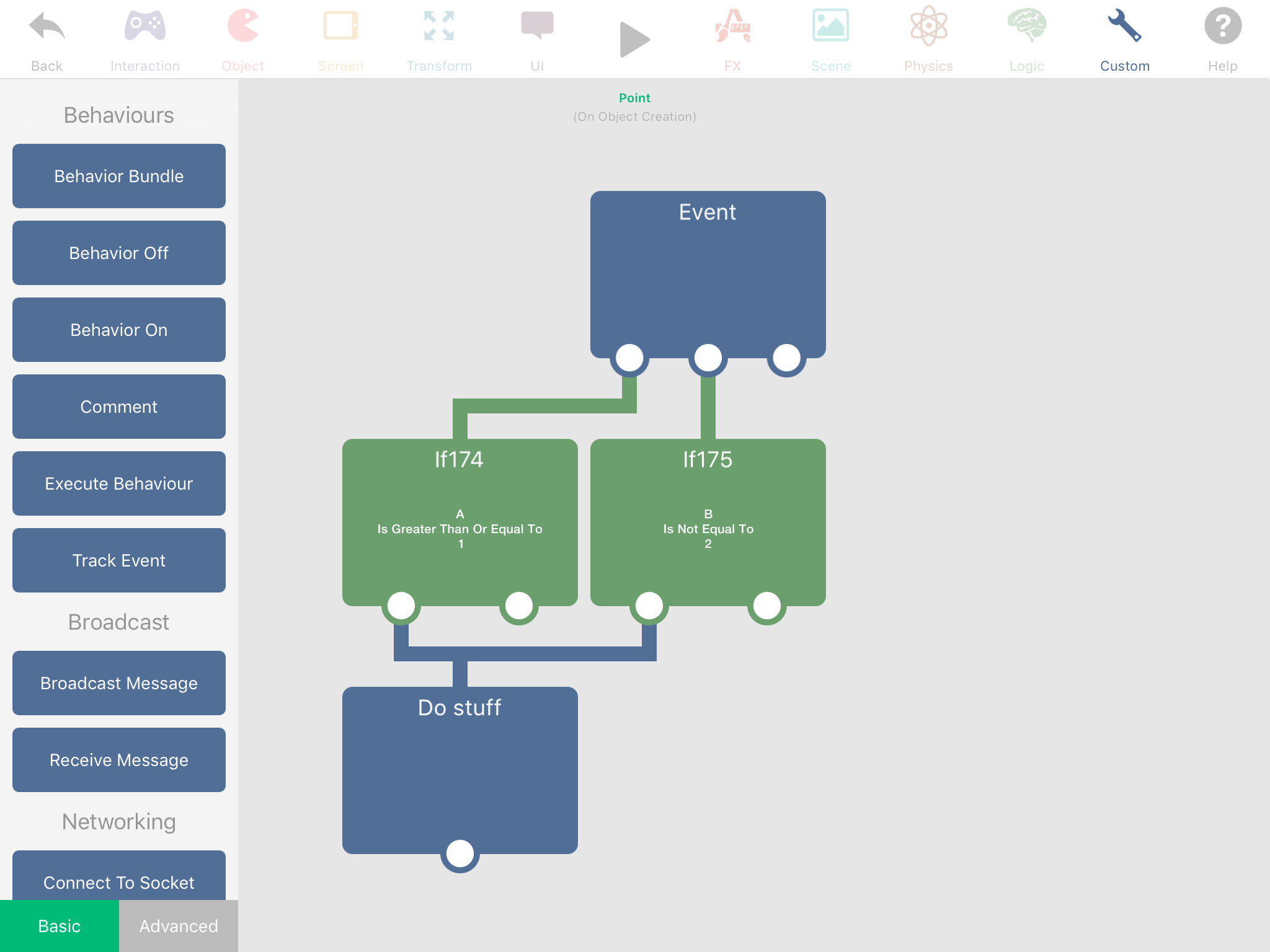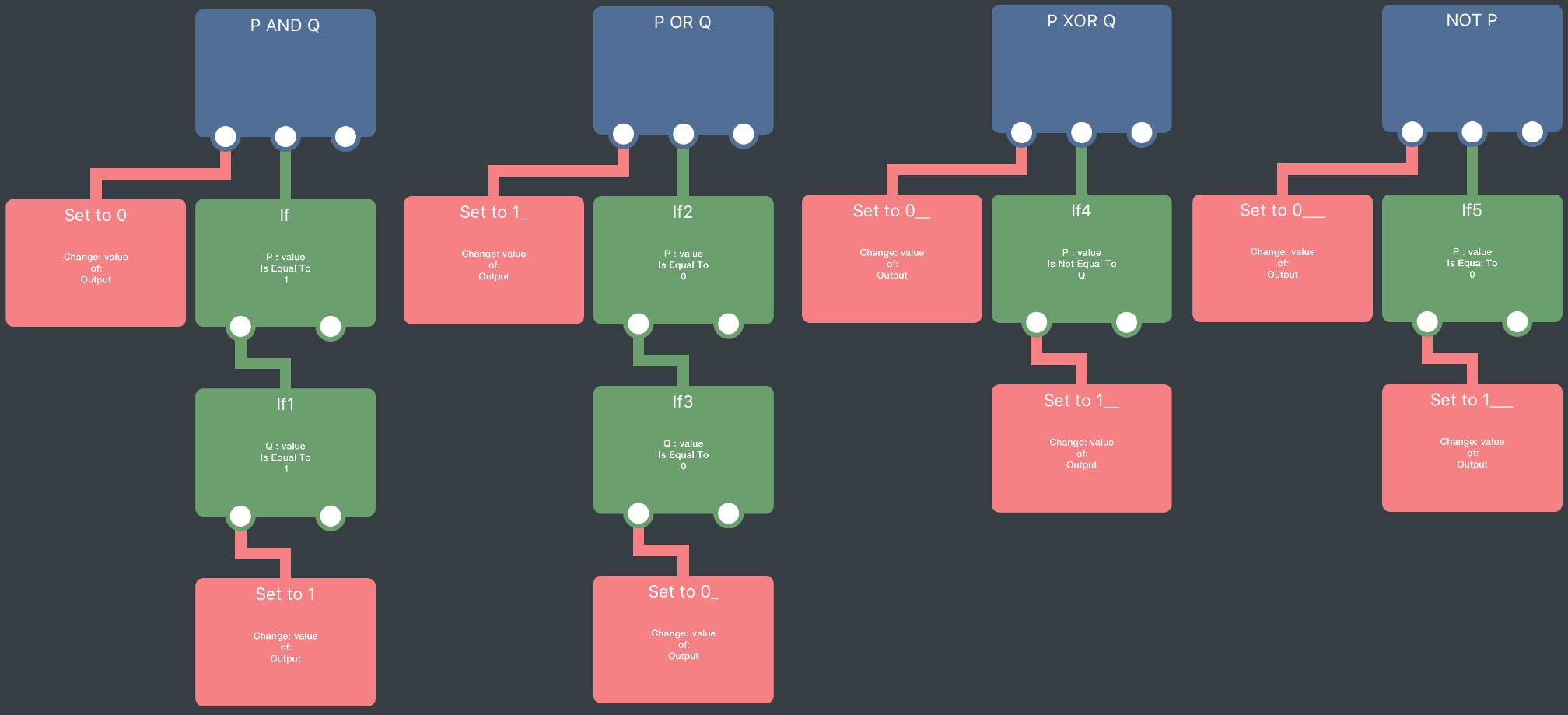How to do logical AND in conditionals?

• If this AND that then jump

... how to do this in hyperPad?

• @Deeeds Have an If stacked on top of another If. The more conditions you have, the longer your If chain is.

•• @Aidan-Oxley What about OR, XOR and NOT?

• @Deeeds For Or, have the two Ifs next to each other underneath the event. I don’t know what XOR is. For not, press the “is equal to” when you tap the If behaviour, there will be a not equal to (≠).

•• @Deeeds

(I'm going to use this to learn the code formatting on the forums)

Like Aidan said, (P AND Q) can be represented by nesting ifs. It can be done as

//# I'm not sure I fully get the syntax for comments
output = 0 //# default to 0 if they aren't both true
if P == 1:
if Q == 1
output = 1 //# set to 1 if they are both true
return output

P OR Q:

output = 1 //# defaults to 1
if P == 0
if Q == 0
output = 0 //# if NOT(P) AND NOT(Q) is true, return 0
return output

(P XOR Q) is the equivalent to ((P OR Q) AND NOT(P AND Q)), but there's an easy shortcut in that it's true when P ≠ Q:

output = 0
if P != Q:
output = 1
return output

Edit: oh yeah, NOT P:

output = 1
if P = 1
output = 0
return output

Edit 2: As hyperPad behaviours:• @Jack8680 INCREDIBLE!!!

THANK YOU!!!!!!!